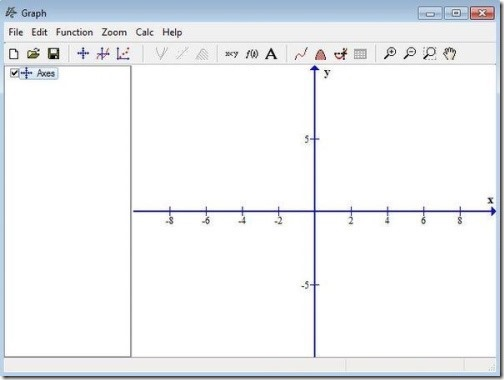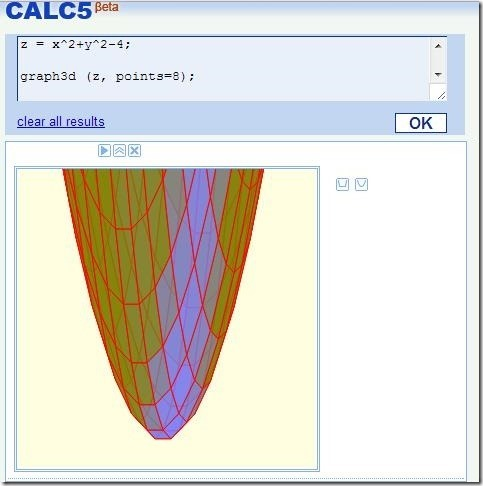Editor Ratings:
User Ratings:
[Total: 0 Average: 0]

Here is a list of 4 free mathematical graph drawing tools that lets you plot graphs simply by typing in the functions, expressions and equations. These free mathematical graph drawing tools comprises of simple and user-friendly interface as it provides you colorful interface along with the ability to type down the desired function or the expression. In addition, these tools lets you save your created graphs in various formats PNG, JPG, PDF, and many more. These tools are apt for both teachers and students as these free mathematical graph drawing tools let you draw graph with ease.

Below I’ve reviewed Graph, Functy, Calc5, and Desmos Graphing Calculator .

## Graph:Graph is a free mathematical graph drawing software for Windows that lets you draw mathematical graphs in a coordinate system. This free mathematical graph drawing software allows you to draw functions for commonly used mathematical functions, linear, trigonometric, etc. It comes with adjustable scale using which you can easily customize the coordinating system for both larger and smaller values. This graph creating software provides you colors and shading styles, which you can use to color the equation or to mark an area related to the function. It lets you calculate the area and the length of the path of the function. This tool lets you customize the function as well as the constants. Not only that, it lets you add text labels to your graphs which comprises of various fonts, images and objects. It lets you save the graph in various formats like JPG, PNG, PDF, SVG, BMP,EMF, and GIF. It provides you the facility to print the created graphs as well. The best part of this free mathematical graph drawing software is that it lets you create animations which you can use to show the changes of the function when any constant value changes.

## Functy:Functy is another free mathematical graph drawing software for Windows that lets you create and render 3D Cartesian and spherical math functions in real time. It allows you to enter the math functions and it quickly displays the view of the plotted graph providing you an option to edit the plotted ranges used in functions, x, y or z. It offers you a viewer where you can rotate, translate, zoom, and explore your functions according to your desire. This free mathematical graph drawing software supports animated functions that uses time variable. It lets you save and export the created graph in PLY format, so that it can be used further with other 3D modeling apps.

## Calc5:Calc5 is a free mathematical graph drawing online tool that lets you plot 2D and 3D graphs simply by typing in the desired mathematical functions. It provides you advanced mathematical graph calculator and supports calculus operations as well. It allows you to make symbolic calculations like differentiation, integration, and more. It also provides you zooming and panning feature, whenever you create 2D graph. You just have to write the desired function and click on ok and your graph is ready. To save the created graph you can take the snapshot via any screen capture tool, as it does not allow you to save the graph.

## Desmos Graphing Calculator:Desmos Graphing Calculator is another free mathematical graph drawing online tool that lets you create simple as well complex graphs simply by typing in the expressions and the equations manually or with the help of rich keypad. It offers you colorful and clutter-free interface where you can draw multiple graphs. This free mathematical graph drawing tool lets you customize the paper format of the graph, depending on your requirement. You need to create an account on this free web service so as to save and share the created graph with your friends and colleagues. It provides you 3 graph saving options, where you can send as email, embed or save the graph as an image in PNG format. Not only that, it also lets you print your graph. It is available as Chrome app also, therefore you can use it right from your Chrome browser.

So, teachers and students try out these free mathematical graph drawing tools and create math graphs. Pen down your feedback and suggestions in the comments section below.

 Editor Ratings: User Ratings:[Total: 0 Average: 0] Free/Paid: Free